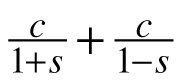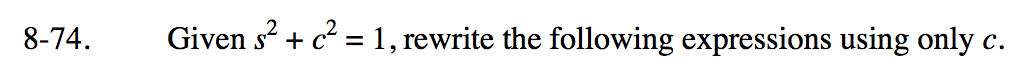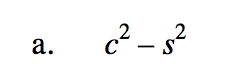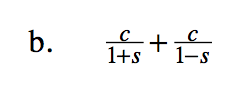### Home > PC3 > Chapter 8 > Lesson 8.2.2 > Problem8-74

8-74.
1. Given s2 + c2 = 1, rewrite the following expressions using only c. Homework Help ✎

1. c2s2

2.Rewrite the given equation for s2.
Then substitute in that expression.$\frac{c}{1+s}\cdot\frac{1-s}{1-s}+\frac{c}{1-s}\cdot\frac{1+s}{1+s}$